Next: Bounding Vectors: The Gate Up: CEM for Gaussian Mixture Previous: Updating the Gates

## Bounding Scalars: The Gate Mixing Proportions

We will update the mixing proportions by holding the experts fixed as well as the gate means and covariances. By taking derivatives of the Q function over only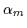we get Equation 7.19.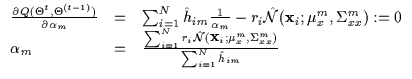(7.19)

The update foris a function of the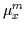and the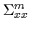. It is possible to keep this equality and use it later as we derive the update rules for the means and the covariances. However, it is quite cumbersome to manipulate analytically if it is maintained as shown above. Thus, we lock the values of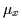and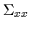at their previous estimates (i.e. at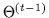) and numerically update the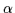mixing proportions.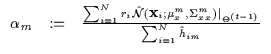(7.20)

Since we are maximizing a bound, the above update rule increases conditional likelihood monotonically. This was also verified with a numerical implementation.Next: Bounding Vectors: The Gate Up: CEM for Gaussian Mixture Previous: Updating the Gates
Tony Jebara
1999-09-15